# Continuous function

In mathematics, a continuous function is, roughly speaking, a function for which sufficiently small changes in the input result in arbitrarily small changes in the output. Otherwise, a function is said to be a discontinuous function. A continuous function with a continuous inverse function is called a homeomorphism.

Continuity of functions is one of the core concepts of topology, which is treated in full generality below. The introductory portion of this article focuses on the special case where the inputs and outputs of functions are real numbers. In addition, this article discusses the definition for the more general case of functions between two metric spaces. In order theory, especially in domain theory, one considers a notion of continuity known as Scott continuity. Other forms of continuity do exist but they are not discussed in this article.

As an example, consider the function h(t), which describes the height of a growing flower at time t. This function is continuous. By contrast, if M(t) denotes the amount of money in a bank account at time t, then the function jumps at each point in time when money is deposited or withdrawn, so the function M(t) is discontinuous.

## History

A form of the epsilon–delta definition of continuity was first given by Bernard Bolzano in 1817. Augustin-Louis Cauchy defined continuity ofas follows: an infinitely small incrementof the independent variable x always produces an infinitely small changeof the dependent variable y (see e.g., Cours d'Analyse, p. 34). Cauchy defined infinitely small quantities in terms of variable quantities, and his definition of continuity closely parallels the infinitesimal definition used today (see microcontinuity). The formal definition and the distinction between pointwise continuity and uniform continuity were first given by Bolzano in the 1830s but the work wasn't published until the 1930s. Like Bolzano, Karl Weierstrass denied continuity of a function at a point c unless it was defined at and on both sides of c, but Édouard Goursat allowed the function to be defined only at and on one side of c, and Camille Jordan allowed it even if the function was defined only at c. All three of those nonequivalent definitions of pointwise continuity are still in use. Eduard Heine provided the first published definition of uniform continuity in 1872, but based these ideas on lectures given by Peter Gustav Lejeune Dirichlet in 1854.

## Real-valued continuous functions

### Definition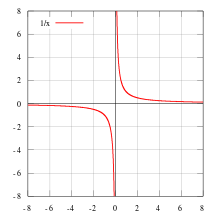The functionis continuous on its whole domain, but is not continuous over the domainbecause it is discontinuous atA function from the set of real numbers to the real numbers can be represented by a graph in the Cartesian plane; such a function is continuous if, roughly speaking, the graph is a single unbroken curve with no "holes" or "jumps". This is not a rigorous definition of continuity since the functionis continuous on its whole domain of(but it is not continuous over the domainbecause it is discontinuous at.)

A function is continuous at a point if it does not have a hole or jump. A “hole” or “jump” in the graph of a function occurs if the value of the function at a point c differs from its limiting value along points that are nearby. Such a point is called a discontinuity. A function is then continuous if it has no holes or jumps: that is, if it is continuous at every point of its domain. Otherwise, a function is discontinuous, at the points where the value of the function differs from its limiting value (if any).

There are several ways to make this definition mathematically rigorous. These definitions are equivalent to one another, so the most convenient definition can be used to determine whether a given function is continuous or not. In the definitions below,is a function defined on a subset I of the set R of real numbers. This subset I is referred to as the domain of f. Some possible choices include I=R, the whole set of real numbers, an open intervalor a closed intervalHere, a and b are real numbers.

#### Definition in terms of limits of functions

The function f is continuous at some point c of its domain if the limit of f(x) as x approaches c through the domain of f exists and is equal to f(c). In mathematical notation, this is written asIn detail this means three conditions: first, f has to be defined at c. Second, the limit on the left hand side of that equation has to exist. Third, the value of this limit must equal f(c).

(We have here assumed that the domain of f does not have any isolated points. For example, an interval or union of intervals has no isolated points.)

#### Definition in terms of neighborhoods

A neighborhood of a point c is a set that contains all points of the domain within some fixed distance of c. Intuitively, a function is continuous at a point c if the range of the restriction of f to a neighborhood of c shrinks to a single point f(c) as the width of the neighborhood shrinks to zero. More precisely, a function f is continuous at a point c of its domain if, for any neighborhoodthere is a neighborhoodsuch thatwhenever.

This definition does only require that the domain and the codomain are topological spaces and is thus the most general definition. From it follows, that the function f is automatically continuous at every isolated point of its domain. As a specific example, every real valued function on the set of integers is continuous.

#### Definition in terms of limits of sequences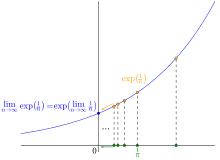The sequence exp(1/n) converges to exp(0)

One can instead require that for any sequenceof points in the domain which converges to c, the corresponding sequenceconverges to f(c). In mathematical notation,#### Weierstrass and Jordan definitions (epsilon–delta) of continuous functions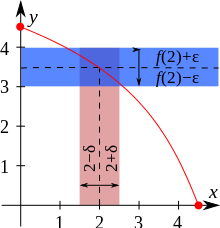Illustration of the ε-δ-definition: for ε=0.5, c=2, the value δ=0.5 satisfies the condition of the definition.

Explicitly including the definition of the limit of a function, we obtain a self-contained definition: Given a function f as above and an element c of the domain I, f is said to be continuous at the point c if the following holds: For any number ε > 0, however small, there exists some number δ > 0 such that for all x in the domain of f with c  δ < x < c + δ, the value of f(x) satisfiesAlternatively written, continuity of f : I  R at c  I means that for every ε > 0 there exists a δ > 0 such that for all x  I,:More intuitively, we can say that if we want to get all the f(x) values to stay in some small neighborhood around f(c), we simply need to choose a small enough neighborhood for the x values around c, and we can do that no matter how small the f(x) neighborhood is; f is then continuous at c.

In modern terms, this is generalized by the definition of continuity of a function with respect to a basis for the topology, here the metric topology.

Weierstrass had required that the interval c  δ < x < c + δ be entirely within the domain I, but Jordan removed that restriction.

#### Definition using oscillation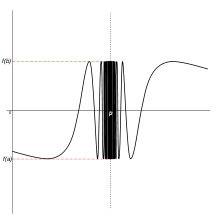The failure of a function to be continuous at a point is quantified by its oscillation.

Continuity can also be defined in terms of oscillation: a function f is continuous at a point x0 if and only if its oscillation at that point is zero; in symbols,A benefit of this definition is that it quantifies discontinuity: the oscillation gives how much the function is discontinuous at a point.

This definition is useful in descriptive set theory to study the set of discontinuities and continuous points – the continuous points are the intersection of the sets where the oscillation is less than ε (hence a Gδ set) – and gives a very quick proof of one direction of the Lebesgue integrability condition.

The oscillation is equivalent to the ε-δ definition by a simple re-arrangement, and by using a limit (lim sup, lim inf) to define oscillation: if (at a given point) for a given ε0 there is no δ that satisfies the ε-δ definition, then the oscillation is at least ε0, and conversely if for every ε there is a desired δ, the oscillation is 0. The oscillation definition can be naturally generalized to maps from a topological space to a metric space.

#### Definition using the hyperreals

Cauchy defined continuity of a function in the following intuitive terms: an infinitesimal change in the independent variable corresponds to an infinitesimal change of the dependent variable (see Cours d'analyse, page 34). Non-standard analysis is a way of making this mathematically rigorous. The real line is augmented by the addition of infinite and infinitesimal numbers to form the hyperreal numbers. In nonstandard analysis, continuity can be defined as follows.

A real-valued function f is continuous at x if its natural extension to the hyperreals has the property that for all infinitesimal dx, f(x+dx) − f(x) is infinitesimal

(see microcontinuity). In other words, an infinitesimal increment of the independent variable always produces to an infinitesimal change of the dependent variable, giving a modern expression to Augustin-Louis Cauchy's definition of continuity.

### Examples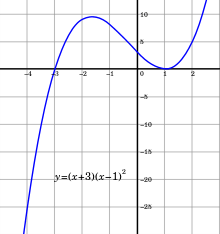The graph of a cubic function has no jumps or holes. The function is continuous.

All polynomial functions, such as f(x) = x3 + x2 - 5x + 3 (pictured), are continuous. This is a consequence of the fact that, given two continuous functionsdefined on the same domain I, then the sum f + g and the product fg of the two functions are continuous (on the same domain I). Moreover, the functionis continuous. (The points where g(x) is zero are discarded, as they are not in the domain of f/g.) For example, the function (pictured)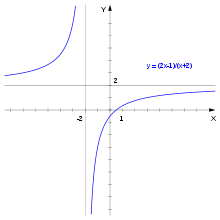The graph of a continuous rational function. The function is not defined for x=−2. The vertical and horizontal lines are asymptotes.

is defined for all real numbers x ≠ −2 and is continuous at every such point. Thus it is a continuous function. The question of continuity at x = −2 does not arise, since x = −2 is not in the domain of f. There is no continuous function F: RR that agrees with f(x) for all x ≠ −2. The sinc function g(x) = (sin x)/x, defined for all x≠0 is continuous at these points. Thus it is a continuous function, too. However, unlike the one of the previous example, this one can be extended to a continuous function on all real numbers, namelysince the limit of g(x), when x approaches 0, is 1. Therefore, the point x=0 is called a removable singularity of g.

Given two continuous functionsthe compositionis continuous.

### Non-examples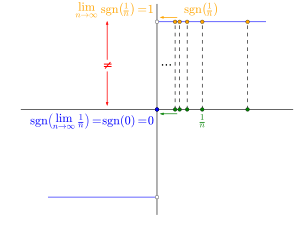Plot of the signum function. It shows that. Thus, the signum function is not continuous at the point 0.

An example of a discontinuous function is the Heaviside step function, defined by. Pick for instance. There is no-neighborhood aroundthat will force all thevalues to be withinof. Intuitively we can think of this type of discontinuity as a sudden jump in function values.

Similarly, the signum or sign functionis discontinuous atbut continuous everywhere else. Yet another example: the functionis continuous everywhere apart from.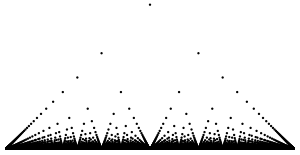Plot of Thomae's function for the domain.is continuous at all irrational numbers and discontinuous at all rational numbers. In a similar vein, Dirichlet's functionis nowhere continuous.

### Properties

#### Intermediate value theorem

The intermediate value theorem is an existence theorem, based on the real number property of completeness, and states:

If the real-valued function f is continuous on the closed interval [a, b] and k is some number between f(a) and f(b), then there is some number c in [a, b] such that f(c) = k.

For example, if a child grows from 1 m to 1.5 m between the ages of two and six years, then, at some time between two and six years of age, the child's height must have been 1.25 m.

As a consequence, if f is continuous on [a, b] and f(a) and f(b) differ in sign, then, at some point c in [a, b], f(c) must equal zero.

#### Extreme value theorem

The extreme value theorem states that if a function f is defined on a closed interval [a,b] (or any closed and bounded set) and is continuous there, then the function attains its maximum, i.e. there exists c  [a,b] with f(c) ≥ f(x) for all x  [a,b]. The same is true of the minimum of f. These statements are not, in general, true if the function is defined on an open interval (a,b) (or any set that is not both closed and bounded), as, for example, the continuous function f(x) = 1/x, defined on the open interval (0,1), does not attain a maximum, being unbounded above.

#### Relation to differentiability and integrabilityis continuous, as can be shown. The converse does not hold: for example, the absolute value functionis everywhere continuous. However, it is not differentiable at x = 0 (but is so everywhere else). Weierstrass's function is also everywhere continuous but nowhere differentiable.

The derivative f′(x) of a differentiable function f(x) need not be continuous. If f′(x) is continuous, f(x) is said to be continuously differentiable. The set of such functions is denoted C1((a, b)). More generally, the set of functions(from an open interval (or open subset of R) Ω to the reals) such that f is n times differentiable and such that the n-th derivative of f is continuous is denoted Cn(Ω). See differentiability class. In the field of computer graphics, these three levels are sometimes called G0 (continuity of position), G1 (continuity of tangency), and G2 (continuity of curvature).

Every continuous functionis integrable (for example in the sense of the Riemann integral). The converse does not hold, as the (integrable, but discontinuous) sign function shows.

#### Pointwise and uniform limits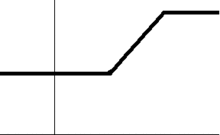A sequence of continuous functions fn(x) whose (pointwise) limit function f(x) is discontinuous. The convergence is not uniform.

Given a sequenceof functions such that the limitexists for all x in I, the resulting function f(x) is referred to as the pointwise limit of the sequence of functions (fn)nN. The pointwise limit function need not be continuous, even if all functions fn are continuous, as the animation at the right shows. However, f is continuous when the sequence converges uniformly, by the uniform convergence theorem. This theorem can be used to show that the exponential functions, logarithms, square root function, trigonometric functions are continuous.

### Directional and semi-continuity

Discontinuous functions may be discontinuous in a restricted way, giving rise to the concept of directional continuity (or right and left continuous functions) and semi-continuity. Roughly speaking, a function is right-continuous if no jump occurs when the limit point is approached from the right. Formally, f is said to be right-continuous at the point c if the following holds: For any number ε > 0 however small, there exists some number δ > 0 such that for all x in the domain with c < x < c + δ, the value of f(x) will satisfyThis is the same condition as for continuous functions, except that it is required to hold for x strictly larger than c only. Requiring it instead for all x with cδ < x < c yields the notion of left-continuous functions. A function is continuous if and only if it is both right-continuous and left-continuous.

A function f is lower semi-continuous if, roughly, any jumps that might occur only go down, but not up. That is, for any ε > 0, there exists some number δ > 0 such that for all x in the domain with |x − c| < δ, the value of f(x) satisfiesThe reverse condition is upper semi-continuity.

## Continuous functions between metric spaces

The concept of continuous real-valued functions can be generalized to functions between metric spaces. A metric space is a set X equipped with a function (called metric) dX, that can be thought of as a measurement of the distance of any two elements in X. Formally, the metric is a functionthat satisfies a number of requirements, notably the triangle inequality. Given two metric spaces (X, dX) and (Y, dY) and a functionthen f is continuous at the point c in X (with respect to the given metrics) if for any positive real number ε, there exists a positive real number δ such that all x in X satisfying dX(x, c) < δ will also satisfy dY(f(x), f(c)) < ε. As in the case of real functions above, this is equivalent to the condition that for every sequence (xn) in X with limit lim xn = c, we have lim f(xn) = f(c). The latter condition can be weakened as follows: f is continuous at the point c if and only if for every convergent sequence (xn) in X with limit c, the sequence (f(xn)) is a Cauchy sequence, and c is in the domain of f.

The set of points at which a function between metric spaces is continuous is a Gδ set – this follows from the ε-δ definition of continuity.

This notion of continuity is applied, for example, in functional analysis. A key statement in this area says that a linear operatorbetween normed vector spaces V and W (which are vector spaces equipped with a compatible norm, denoted ||x||) is continuous if and only if it is bounded, that is, there is a constant K such thatfor all x in V.

### Uniform, Hölder and Lipschitz continuity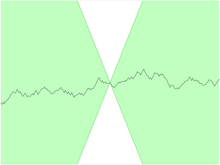For a Lipschitz continuous function, there is a double cone (shown in white) whose vertex can be translated along the graph, so that the graph always remains entirely outside the cone.

The concept of continuity for functions between metric spaces can be strengthened in various ways by limiting the way δ depends on ε and c in the definition above. Intuitively, a function f as above is uniformly continuous if the δ does not depend on the point c. More precisely, it is required that for every real number ε > 0 there exists δ > 0 such that for every c, b  X with dX(b, c) < δ, we have that dY(f(b), f(c)) < ε. Thus, any uniformly continuous function is continuous. The converse does not hold in general, but holds when the domain space X is compact. Uniformly continuous maps can be defined in the more general situation of uniform spaces.

A function is Hölder continuous with exponent α (a real number) if there is a constant K such that for all b and c in X, the inequalityholds. Any Hölder continuous function is uniformly continuous. The particular case α = 1 is referred to as Lipschitz continuity. That is, a function is Lipschitz continuous if there is a constant K such that the inequalityholds for any b, c in X. The Lipschitz condition occurs, for example, in the Picard–Lindelöf theorem concerning the solutions of ordinary differential equations.

## Continuous functions between topological spaces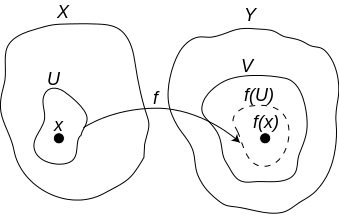Another, more abstract, notion of continuity is continuity of functions between topological spaces in which there generally is no formal notion of distance, as there is in the case of metric spaces. A topological space is a set X together with a topology on X, which is a set of subsets of X satisfying a few requirements with respect to their unions and intersections that generalize the properties of the open balls in metric spaces while still allowing to talk about the neighbourhoods of a given point. The elements of a topology are called open subsets of X (with respect to the topology).

A functionbetween two topological spaces X and Y is continuous if for every open set VY, the inverse imageis an open subset of X. That is, f is a function between the sets X and Y (not on the elements of the topology TX), but the continuity of f depends on the topologies used on X and Y.

This is equivalent to the condition that the preimages of the closed sets (which are the complements of the open subsets) in Y are closed in X.

An extreme example: if a set X is given the discrete topology (in which every subset is open), all functionsto any topological space T are continuous. On the other hand, if X is equipped with the indiscrete topology (in which the only open subsets are the empty set and X) and the space T set is at least T0, then the only continuous functions are the constant functions. Conversely, any function whose range is indiscrete is continuous.

### Alternative definitions

Several equivalent definitions for a topological structure exist and thus there are several equivalent ways to define a continuous function.

#### Neighborhood definition

Continuity for functions between topological spacesandat a point may be defined using the notion of neighborhoods: A functionis continuous at a pointif and only if for any neighborhood of its imagethe preimage is again a neighborhood of that point:According to the property that neighborhood systems being upper sets this can be restated as follows:The second one being a restatement involving the image rather than the preimage.
Literally, this means no matter how small the neighborhood is chosen one can always find a neighborhood mapped into it.

Besides, there's a simplification involving only open neighborhoods. In fact, they're equivalent:The second one again being a restatement using images rather than preimages.

If X and Y are metric spaces, it is equivalent to consider the neighborhood system of open balls centered at x and f(x) instead of all neighborhoods. This gives back the above δ-ε definition of continuity in the context of metric spaces.

In general topological spaces, there is no notion of nearness or distance. If however the target space is a Hausdorff space, it is still true that f is continuous at a if and only if the limit of f as x approaches a is f(a).

At an isolated point, every function is continuous.

#### Sequences and nets

In several contexts, the topology of a space is conveniently specified in terms of limit points. In many instances, this is accomplished by specifying when a point is the limit of a sequence, but for some spaces that are too large in some sense, one specifies also when a point is the limit of more general sets of points indexed by a directed set, known as nets. A function is (Heine-)continuous only if it takes limits of sequences to limits of sequences. In the former case, preservation of limits is also sufficient; in the latter, a function may preserve all limits of sequences yet still fail to be continuous, and preservation of nets is a necessary and sufficient condition.

In detail, a function f: XY is sequentially continuous if whenever a sequence (xn) in X converges to a limit x, the sequence (f(xn)) converges to f(x). Thus sequentially continuous functions "preserve sequential limits". Every continuous function is sequentially continuous. If X is a first-countable space and countable choice holds, then the converse also holds: any function preserving sequential limits is continuous. In particular, if X is a metric space, sequential continuity and continuity are equivalent. For non first-countable spaces, sequential continuity might be strictly weaker than continuity. (The spaces for which the two properties are equivalent are called sequential spaces.) This motivates the consideration of nets instead of sequences in general topological spaces. Continuous functions preserve limits of nets, and in fact this property characterizes continuous functions.

#### Closure operator definition

Instead of specifying the open subsets of a topological space, the topology can also be determined by a closure operator (denoted cl) which assigns to any subset AX its closure, or an interior operator (denoted int), which assigns to any subset A of X its interior. In these terms, a functionbetween topological spaces is continuous in the sense above if and only if for all subsets A of XThat is to say, given any element x of X that is in the closure of any subset A, f(x) belongs to the closure of f(A). This is equivalent to the requirement that for all subsets A' of X'Moreover,is continuous if and only iffor any subset A' of Y.

### Properties

If f: XY and g: YZ are continuous, then so is the composition gf: XZ. If f: XY is continuous and

The possible topologies on a fixed set X are partially ordered: a topology τ1 is said to be coarser than another topology τ2 (notation: τ1 ⊆ τ2) if every open subset with respect to τ1 is also open with respect to τ2. Then, the identity map

idX: (X, τ2) → (X, τ1)

is continuous if and only if τ1 ⊆ τ2 (see also comparison of topologies). More generally, a continuous functionstays continuous if the topology τY is replaced by a coarser topology and/or τX is replaced by a finer topology.

### Homeomorphisms

Symmetric to the concept of a continuous map is an open map, for which images of open sets are open. In fact, if an open map f has an inverse function, that inverse is continuous, and if a continuous map g has an inverse, that inverse is open. Given a bijective function f between two topological spaces, the inverse function f−1 need not be continuous. A bijective continuous function with continuous inverse function is called a homeomorphism.

If a continuous bijection has as its domain a compact space and its codomain is Hausdorff, then it is a homeomorphism.

### Defining topologies via continuous functions

Given a functionwhere X is a topological space and S is a set (without a specified topology), the final topology on S is defined by letting the open sets of S be those subsets A of S for which f−1(A) is open in X. If S has an existing topology, f is continuous with respect to this topology if and only if the existing topology is coarser than the final topology on S. Thus the final topology can be characterized as the finest topology on S that makes f continuous. If f is surjective, this topology is canonically identified with the quotient topology under the equivalence relation defined by f.

Dually, for a function f from a set S to a topological space, the initial topology on S has as open subsets A of S those subsets for which f(A) is open in X. If S has an existing topology, f is continuous with respect to this topology if and only if the existing topology is finer than the initial topology on S. Thus the initial topology can be characterized as the coarsest topology on S that makes f continuous. If f is injective, this topology is canonically identified with the subspace topology of S, viewed as a subset of X.

More generally, given a set S, specifying the set of continuous functionsinto all topological spaces X defines a topology. Dually, a similar idea can be applied to mapsThis is an instance of a universal property.

Various other mathematical domains use the concept of continuity in different, but related meanings. For example, in order theory, an order-preserving function f: XY between particular types of partially ordered sets X and Y is continuous if for each directed subset A of X, we have sup(f(A)) = f(sup(A)). Here sup is the supremum with respect to the orderings in X and Y, respectively. This notion of continuity is the same as topological continuity when the partially ordered sets are given the Scott topology.between two categories is called continuous, if it commutes with small limits. That is to say,for any small (i.e., indexed by a set I, as opposed to a class) diagram of objects in.

A continuity space is a generalization of metric spaces and posets, which uses the concept of quantales, and that can be used to unify the notions of metric spaces and domains.Wikimedia Commons has media related to Continuity (functions).
1. Bolzano, Bernard (1817), Rein analytischer Beweis des Lehrsatzes dass zwischen je zwey Werthen, die ein entgegengesetztes Resultat gewaehren, wenigstens eine reele Wurzel der Gleichung liege, Prague: Haase
2. Dugac, Pierre (1973), "Eléments d'Analyse de Karl Weierstrass", Archive for History of Exact Sciences, 10: 41–176, doi:10.1007/bf00343406
3. Goursat, E. (1904), A course in mathematical analysis, Boston: Ginn, p. 2
4. Jordan, M.C. (1893), Cours d'analyse de l'École polytechnique, 1 (2nd ed.), Paris: Gauthier-Villars, p. 46
5. Harper, J.F. (2016), "Defining continuity of real functions of real variables", BSHM Bulletin: Journal of the British Society for the History of Mathematics: 1–16, doi:10.1080/17498430.2015.1116053
6. Rusnock, P.; Kerr-Lawson, A. (2005), "Bolzano and uniform continuity", Historia Mathematica, 32 (3): 303–311, doi:10.1016/j.hm.2004.11.003
7. Speck, Jared (2014). "Continuity and Discontinuity" (PDF). MIT Math. p. 3. Retrieved 2016-09-02. Example 5. The function 1/x is continuous on (0, ∞) and on (−∞, 0), i.e., for x > 0 and for x < 0, in other words, at every point in its domain. However, it is not a continuous function since its domain is not an interval. It has a single point of discontinuity, namely x = 0, and it has an infinite discontinuity there.
8. Lang, Serge (1997), Undergraduate analysis, Undergraduate Texts in Mathematics (2nd ed.), Berlin, New York: Springer-Verlag, ISBN 978-0-387-94841-6, section II.4
9. Introduction to Real Analysis, updated April 2010, William F. Trench, Theorem 3.5.2, p. 172
10. Introduction to Real Analysis, updated April 2010, William F. Trench, 3.5 "A More Advanced Look at the Existence of the Proper Riemann Integral", pp. 171–177
11. "Elementary Calculus". wisc.edu.
12. Gaal, Steven A. (2009), Point set topology, New York: Dover Publications, ISBN 978-0-486-47222-5, section IV.10
13. Searcóid, Mícheál Ó (2006), Metric spaces, Springer undergraduate mathematics series, Berlin, New York: Springer-Verlag, ISBN 978-1-84628-369-7, section 9.4
14. Goubault-Larrecq, Jean (2013). Non-Hausdorff Topology and Domain Theory: Selected Topics in Point-Set Topology. Cambridge University Press. ISBN 1107034132.
15. Gierz, G.; Hofmann, K. H.; Keimel, K.; Lawson, J. D.; Mislove, M. W.; Scott, D. S. (2003). Continuous Lattices and Domains. Encyclopedia of Mathematics and its Applications. 93. Cambridge University Press. ISBN 0521803381.
16. Flagg, R. C. (1997). "Quantales and continuity spaces". Algebra Universalis. CiteSeerX 10.1.1.48.851.
17. Kopperman, R. (1988). "All topologies come from generalized metrics". American Mathematical Monthly. 95 (2): 89–97. doi:10.2307/2323060.
18. Flagg, B.; Kopperman, R. (1997). "Continuity spaces: Reconciling domains and metric spaces". Theoretical Computer Science. 177 (1): 111–138. doi:10.1016/S0304-3975(97)00236-3.